# Volumes of Prisms and Cylinders Find volumes of

• Slides: 15Volumes of Prisms and Cylinders – Find volumes of prisms. – Find volumes of cylinders.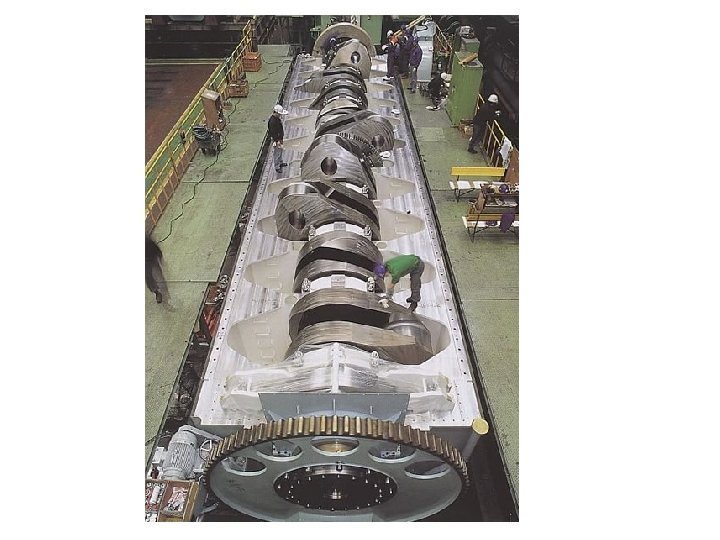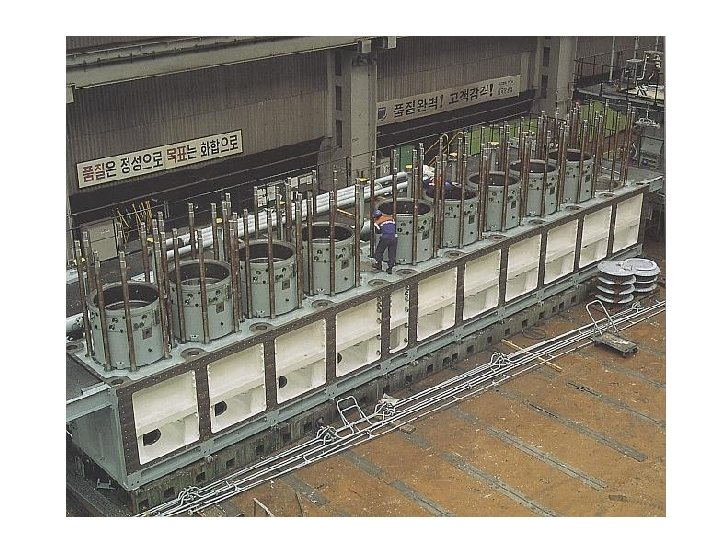Wartsila-Sulzer RTA 96 -C turbocharged two-stroke diesel engine Total engine weight: 2300 tons Maximum power: 108, 920 hp at 102 rpm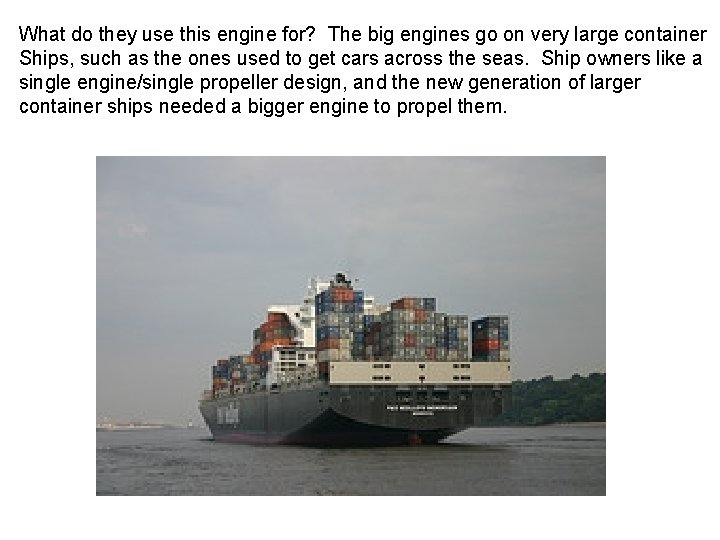What do they use this engine for? The big engines go on very large container Ships, such as the ones used to get cars across the seas. Ship owners like a single engine/single propeller design, and the new generation of larger container ships needed a bigger engine to propel them.VOLUMES OF PRISMS The volume of a figure is the measure of the amount of space that the figure encloses. Volume is measured in cubic units.Key Concept Volume of a Prism If a prism has a volume of V cubic units, a height of h units, and each base has a area of B square units, then V = Bh h Area of base = BExample 1 Volume of a Triangular Prism Find the volume of the triangular prism. 17 cm 8 cm 13 cm a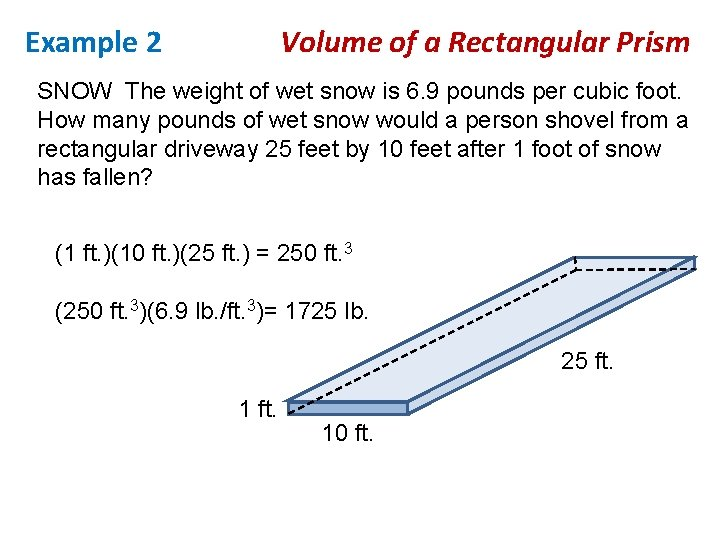Example 2 Volume of a Rectangular Prism SNOW The weight of wet snow is 6. 9 pounds per cubic foot. How many pounds of wet snow would a person shovel from a rectangular driveway 25 feet by 10 feet after 1 foot of snow has fallen? (1 ft. )(10 ft. )(25 ft. ) = 250 ft. 3 (250 ft. 3)(6. 9 lb. /ft. 3)= 1725 lb. 25 ft. 1 ft. 10 ft.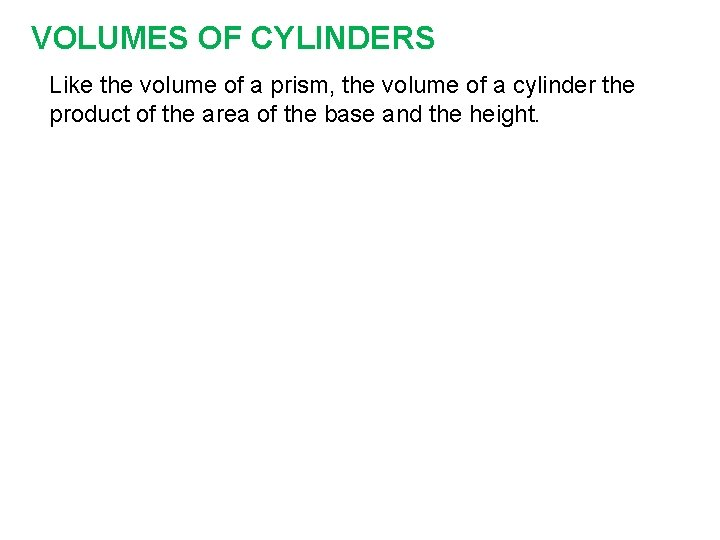VOLUMES OF CYLINDERS Like the volume of a prism, the volume of a cylinder the product of the area of the base and the height.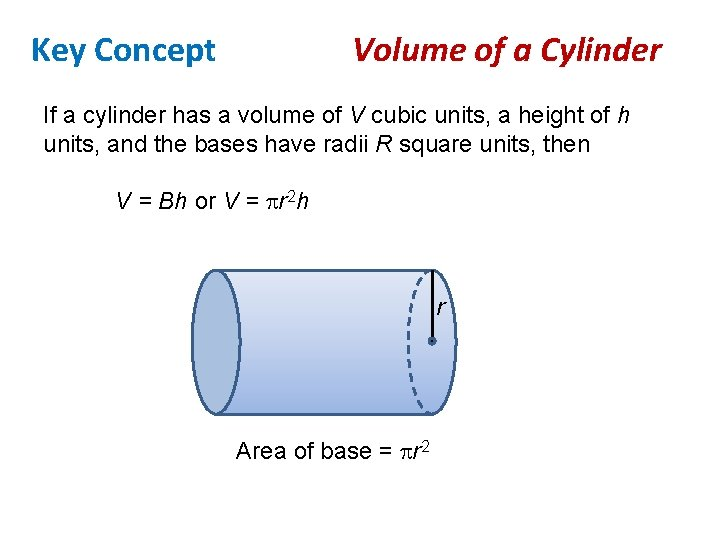Key Concept Volume of a Cylinder If a cylinder has a volume of V cubic units, a height of h units, and the bases have radii R square units, then V = Bh or V = r 2 h r Area of base = r 2Example 3 Volume of a Cylinder Find the volume of the cylinder. 12. 4 m 4. 6 mExample 4 Volume of a Cylinder Find the volume of the cylinder. 5 in. 13 in.Key Concept Cavalieri’s Principle If two solids have the same height and the same crosssectional area at the same level, then they have the same volume.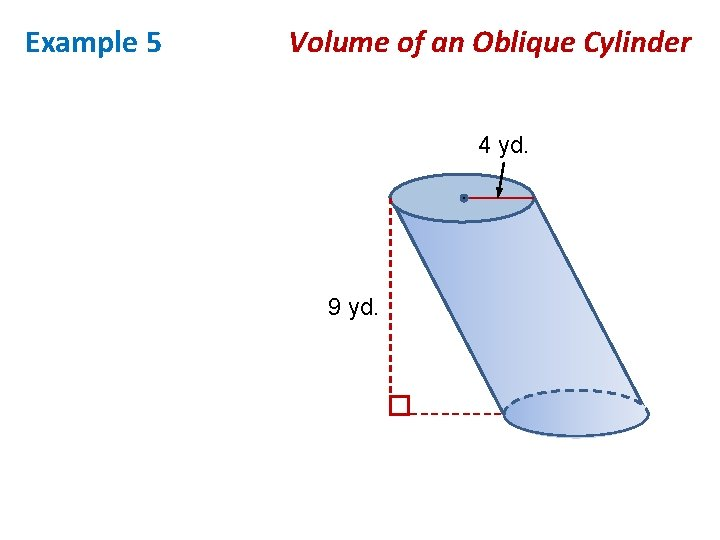Example 5 Volume of an Oblique Cylinder 4 yd. 9 yd.